IMO Shortlist 2014 problem N5

Kvaliteta:
Avg: 0,0
Težina:
Avg: 8,0

Find all triples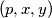$(p, x, y)$ consisting of a prime number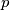$p$ and two positive integers$x$ and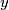$y$ such that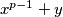$x^{p-1} + y$ and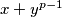$x + y^{p-1}$ are both powers of$p$.

(Belgium)

Izvor: https://www.imo-official.org/problems/IMO2014SL.pdf